# Approximation of a differential boundary value problem by difference boundary value problems

(diff) ← Older revision | Latest revision (diff) | Newer revision → (diff)

An approximation of a differential equation and its boundary conditions by a system of finite (usually algebraic) equations giving the values of the unknown function on some grid, which is subsequently made more exact by making the parameter of the finite-difference problem (the step of the grid, the mesh) tend to zero.

Consider the computation of a function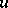which belongs to a linear normed spaceof functions defined in a given domain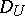with boundary, and which is the solution of the differential boundary value problem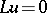,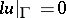, whereis a differential equation, whileis the set of boundary conditions. Let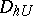be a grid (cf. Approximation of a differential operator by difference operators) and let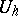be the normed linear space of functions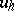defined on this grid. Let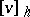be a table of values of the function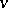at the points of. A norm is introduced intoso that the equalityis valid for any function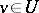. The problem of computing the solutionis replaced by a certain problemfor the approximate computation of the tableof values ofat the points of. Here,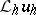is a certain set of (non-differential) equations for the values of the grid function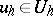.

Let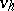be an arbitrary function of, let, and let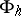be the normed linear space to which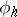belongs for any. One says that the problemis a finite-difference approximation of orderof the differential boundary value problem,, on the space of solutionsof the latter ifThe actual construction of the systeminvolves a separate construction of its two subsystems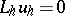and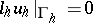. Forone uses the difference approximations of a differential equation (cf. Approximation of a differential equation by difference equations). The complementary equationsare constructed using the boundary conditions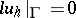.

An approximation such as has just been described never ensures  that the solutionof the finite-difference problem converges to the exact solution, i.e. that the equalityis valid, no matter how the norms inandhave been chosen.

The additional condition, the fulfillment of which in fact ensures convergence, is stability , , which must be displayed by the finite-difference problem. This problem is called stable if there exist numbers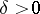and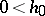such that the equationhas a unique solution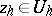for any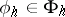,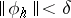,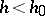, and if this solution satisfies the inequality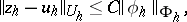where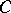is a constant not depending on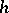or on the perturbationof the right-hand side, whileis a solution of the unperturbed problem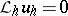. If a solutionof the differential problem exists, while the finite-difference problemapproximates the differential problem on solutionsof orderand is stable, then one has convergence of the same order, i.e.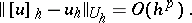For instance, the problem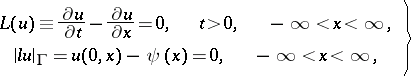(1)

whereis a given function with a bounded second-order derivative, can be approximated, for a natural definition of the norms, by the finite-difference problem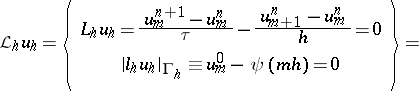(2)where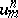is the value ofat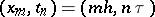,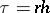,. If the norm of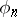is taken to be the upper bound of the moduli of the right-hand sides of the equations which constitute the system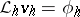,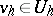, then the approximation of problem (1) by problem (2) on solutionsis of the first order. If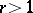, there is no convergence, whatever the norm. If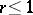and the norm is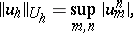the problem is stable, so that there is convergence , :The replacement of differential problems by difference problems is one of the most universal methods for the approximate computation of solutions of differential boundary value problems on a computer .

The replacement of differential problems by their difference analogues started in the works ,  and , and is sometimes employed to prove that the differential problem is in fact solvable. This is done as follows. It is proved that the set of solutionsof the difference analogue of the differential boundary value problem is compact with respect to, after which a proof is given that a solutionof the differential boundary value problem is the limit of a subsequencewhich converges as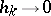. If this solution is known to be unique, then not only the subsequence, but also the entire set ofconverges to the solutionas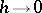.

How to Cite This Entry:
Approximation of a differential boundary value problem by difference boundary value problems. Encyclopedia of Mathematics. URL: http://encyclopediaofmath.org/index.php?title=Approximation_of_a_differential_boundary_value_problem_by_difference_boundary_value_problems&oldid=13955
This article was adapted from an original article by V.S. Ryaben'kii (originator), which appeared in Encyclopedia of Mathematics - ISBN 1402006098. See original article